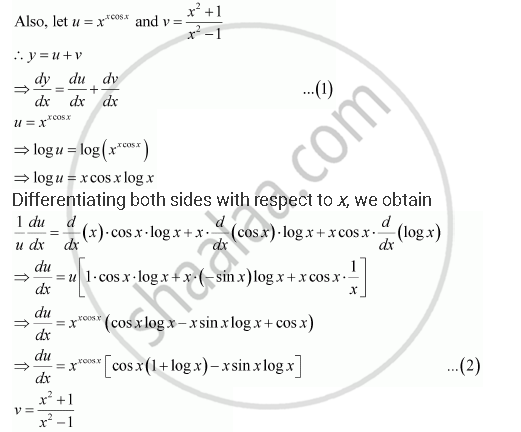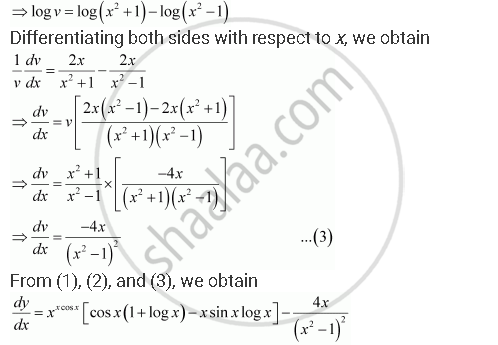Share

# Differentiate the Function with Respect To X. X^(Xcosx) + (X^2 + 1)/(X^2 -1) - CBSE (Science) Class 12 - Mathematics

#### Question

Differentiate the function with respect to x.

x^(xcosx) + (x^2 + 1)/(x^2 -1)

#### Solution

Let y  = x^(xcosx) + (x^2 + 1)/(x^2 -1)Is there an error in this question or solution?

#### APPEARS IN

NCERT Solution for Mathematics Textbook for Class 12 (2018 to Current)
Chapter 5: Continuity and Differentiability
Q: 10 | Page no. 178

#### Video TutorialsVIEW ALL 

Solution Differentiate the Function with Respect To X. X^(Xcosx) + (X^2 + 1)/(X^2 -1) Concept: Logarithmic Differentiation.
S